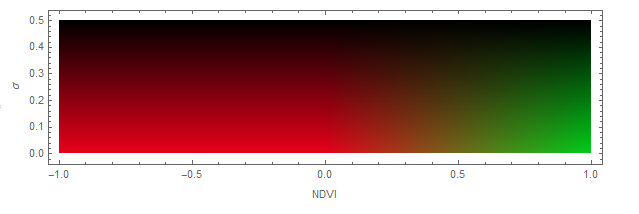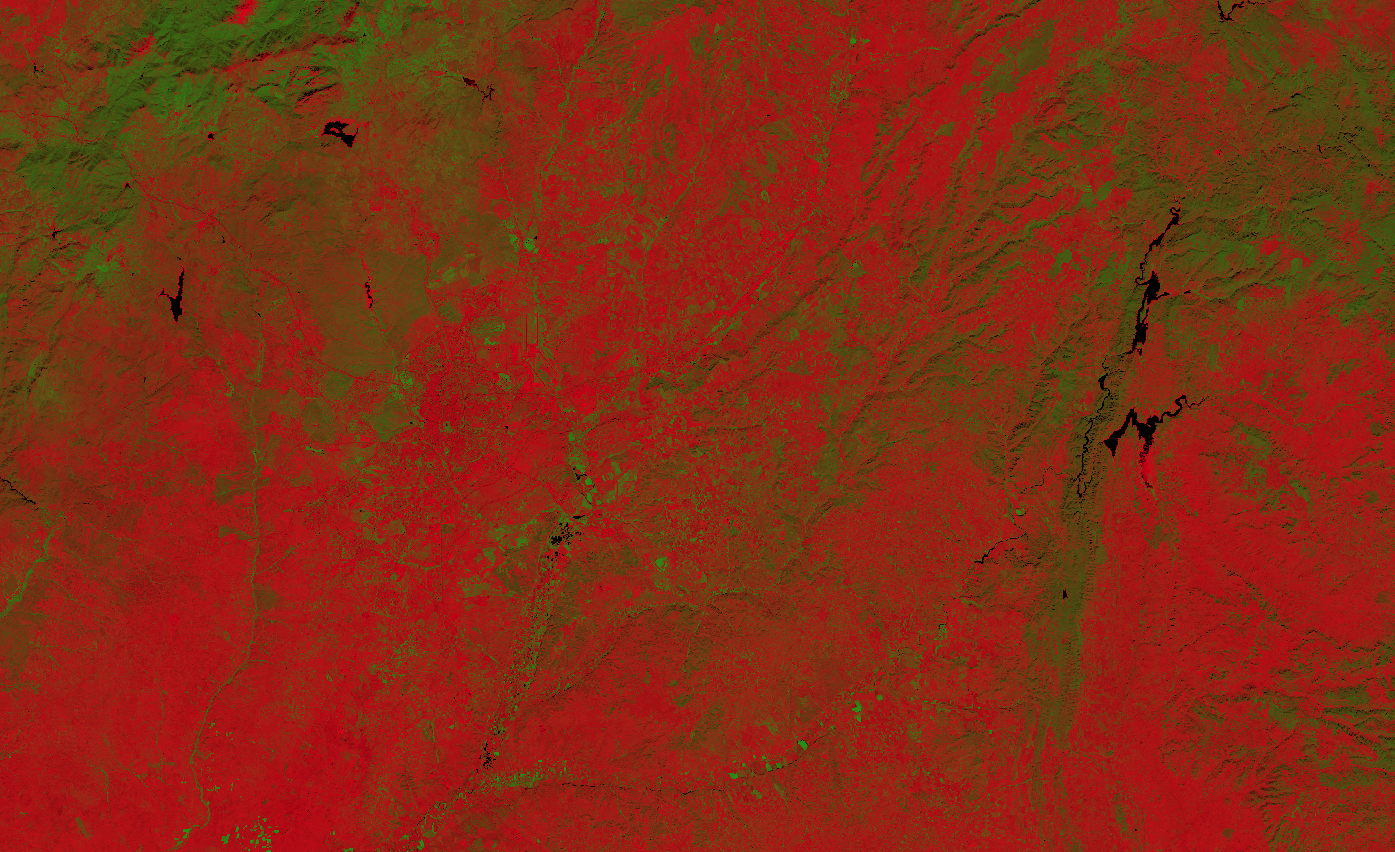# Normalized difference vegetation index with uncertainty

## Basic information

• Bands used to calculate NDVI index: B4, B8
• Bands used by the script: B2, B3, B4, B8

## General description

This script allows you to visually interpret how the normalized density vegetation index (NDVI)  is affected by the uncertainties in detector reflectances of the L1C products.

Since NDVI is defined as a ratio of difference over sum of bands 8 and 4 (near infrared and red):
$$NDVI := \mathtt{Index}(B8,B4) = \frac{B8-B4}{B8+B4}.$$

the uncertainty propagation  gives us the uncertainty of the index itself as

$$\Delta_{NDVI} := \frac{\sqrt{B8^2 \Delta_{B4}^2 + B4^2 \Delta_{B8}^2 - 2B4 B8 \Delta_{B4B8}}}{(B8+B4)^2}$$.

where $\Delta_{B4}$ and $\Delta_{B8}$ are uncertainties of red and near infrared bands respectively (reported by ESA to be 0.02 and 0.03). We left out the mixed part $\Delta_{B4B8}$ as if the two uncertainties were not correlated.

The script encodes the uncertainty with darkness, as can be seen in following figure## Description of representative images

NDVI with uncertainty of Madrid. Acquired on 10.26.2019.Wikipedia, Normalized Difference Vegetation Index . Accessed on October 4th 2017.
 Sentinel-Hub, Ad hoc testing of algorithms globally. Accessed October 10th 2017.
 Wikipedia, Propagation of uncertainty. Accessed October 10th 2017.